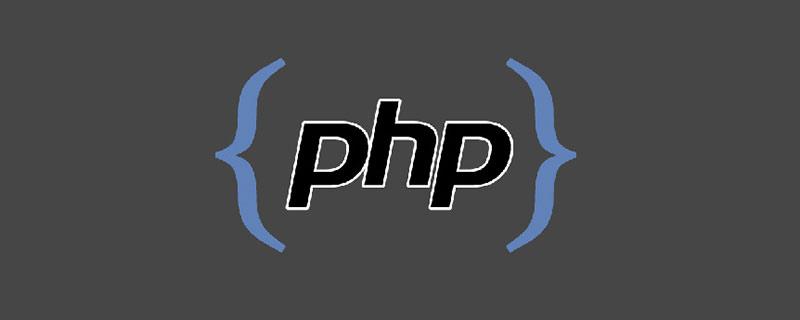php中用foreach改变数组的值的问题

“foreach 语法结构提供了遍历数组的简单方式。foreach 仅能够应用于数组和对象，如果尝试应用于其他数据类型的变量，或者未初始化的变量将发出错误信息。有两种语法：

```foreach (array_expression as \$value)
statement
foreach (array_expression as \$key => \$value)
statement```

```foreach(\$array as \$k => \$v){
\$v = 1;
}```

```foreach(\$array as \$k => \$v){
\$array[\$k] = 1;
}```

```foreach(\$array as &\$v){
\$v = 1;
}
unset(\$v); // 最后取消掉引用```

php如何使用foreach修改数组